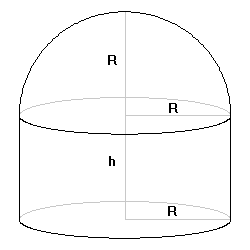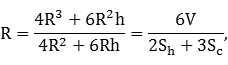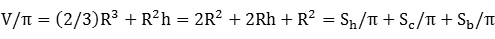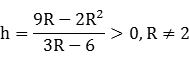Equable Hemispherical Cylinder

EQUABLE HEMISPHERICAL CYLINDER

Balmoral Software

Solutions: 1Consider the "hemispherical cylinder" consisting of a hemisphere of radius R surmounting a cylinder of the same radius and of height h. There is one such polytope that is equable, with integer-valued surface area sections.

The total volume of the hemisphere and cylinder is V, where

 V/π = (2/3)R3 + R2h 
The surface area of the hemisphere is Sh, where
 Sh/π = 2R2 
The lateral surface area of the cylinder is Sc, where
 Sc/π = 2Rh 
The surface area of the cylinder base is Sb, where
 Sb/π = R2 
The above four quantities are integers by convention. From ,  and , we haveso R is rational. By , R2 is an integer, and it follows that R is an integer. The equability requirement iswhich reduces toThe numerator and denominator must have the same sign, so
2 < R < 9/2

R = 3 or R = 4

In the latter case, h = 2/3 by  but Sc/π = 2Rh = 16/3 is not an integer. However, if R = 3, then h = 3 and 2Rh = 18. Therefore, the only equable hemispherical cylinder has a radius and height of 3, and volume and surface area equal to 45 π. It is a solid of revolution of a non-equable closed shape consisting of a 3 x 3 square appended to a quarter-circle of radius 3, having perimeter 3π/2 + 12 = 16.71 and area 9π/4 + 9 = 16.07.